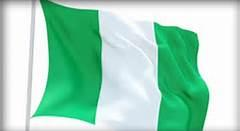# Geography Quiz / Populous Countries

Random Geography Quiz

## Can you name the Populous Countries?

#### bydubem  Plays Quiz not verified by Sporcle

Score
0/20
Timer
02:00
Capital = Beijing
Population = 1.379 billion
Capital = New Delhi
Population = 1.326 billion
Capital = Washington D.C
Population = 324.029 million
Capital = Jakarta
Population = 260.641 million
Capital = Brasilia
Population = 209.629 million
Population = 192.552 million
Capital = Abuja
Population = 186.63 million
Capital = Dhaka
Population = 162.756 million
Capital = Moscow
Population = 146.355 million
Capital = Mexico City
Population = 128.646 million
Capital = Tokyo
Population = 126.345 million
Capital = Manila
Population = 102.123 million
Population = 101.651 million
Capital = Hanoi
Population = 94.362 million
Capital = Cairo
Think
Capital = Berlin
Think
Capital = Tehran
Think
Capital = Ankara
Think
Capital = Kinshasa
Think
Capital = Bangkok
Think

## From the Vault See Another

5 in 15: Harry Potter Characters II
This quiz moves faster than a golden snitch.

### Extras

Created May 9, 2016